# Colleges with the lowest SAT scores in Kansas

Top 10 colleges in Kansas with the lowest SAT scores
Looking for the colleges with the lowest SAT scores in Kansas? Well you're in luck! We've compiled a national college database and have created a list of the top 10 universities with the lowest SAT scores in Kansas below. If you are not a good test taker or worried about your test scores, this list is for you. These are the schools whose applicants had the lowest average SAT scores in Kansas, which means that you can get into these colleges with a lower SAT score. We also include each college's ACT scores and acceptance rate so that you can see where you would have the easiest time getting in. Read on to find out more.

## Hesston College SAT scores

The average SAT score for Hesston College is 830.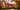The average SAT score of 830 breaks down into:

• SAT math: 400

The average ACT score for Hesston College is 15 and their acceptance rate is 100%.

## Emporia State University SAT scores

The average SAT score for Emporia State University is 1060.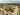The average SAT score of 1060 breaks down into:

• SAT math: 540

The average ACT score for Emporia State University is 22 and their acceptance rate is 82.8%.

## University of Saint Mary SAT scores

The average SAT score for University of Saint Mary is 1070.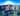The average SAT score of 1070 breaks down into:

• SAT math: 530

The average ACT score for University of Saint Mary is 22 and their acceptance rate is 61.3%.

## Fort Hays State University SAT scores

The average SAT score for Fort Hays State University is 1080.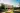The average SAT score of 1080 breaks down into:

• SAT math: 540

The average ACT score for Fort Hays State University is 21 and their acceptance rate is 89%.

## McPherson College SAT scores

The average SAT score for McPherson College is 1090.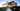The average SAT score of 1090 breaks down into:

• SAT math: 550

The average ACT score for McPherson College is 22 and their acceptance rate is 77.1%.

## Pittsburg State University SAT scores

The average SAT score for Pittsburg State University is 1110.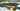The average SAT score of 1110 breaks down into:

• SAT math: 555

The average ACT score for Pittsburg State University is 22 and their acceptance rate is 87.2%.

## Washburn University SAT scores

The average SAT score for Washburn University is 1110.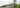The average SAT score of 1110 breaks down into:

• SAT math: 555

The average ACT score for Washburn University is 22 and their acceptance rate is 98.8%.

## Newman University SAT scores

The average SAT score for Newman University is 1140.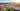The average SAT score of 1140 breaks down into:

• SAT math: 550

The average ACT score for Newman University is 23 and their acceptance rate is 63.8%.

## Wichita State University SAT scores

The average SAT score for Wichita State University is 1140.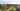The average SAT score of 1140 breaks down into:

• SAT math: 580

The average ACT score for Wichita State University is 23 and their acceptance rate is 97.1%.

## Kansas State University SAT scores

The average SAT score for Kansas State University is 1160.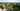The average SAT score of 1160 breaks down into:

• SAT math: 580